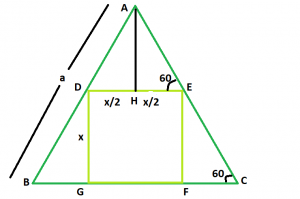# Biggest Square that can be inscribed within an Equilateral triangle

Given here is an equilateral triangle of side length a. The task is to find the side of the biggest square that can be inscribed within it.

Examples:

```Input: a = 5
Output: 2.32

Input: a = 7
Output: 3.248
```

## Recommended: Please try your approach on {IDE} first, before moving on to the solution.Approach: Let the side of the square be x.
Now, AH is perpendicular to DE.
DE is parallel to BC, So, angle AED = angle ACB = 60

```In triangle EFC,
=> Sin60 = x/ EC
=> √3 / 2 = x/EC
=> EC = 2x/√3
In triangle AHE,
=> Cos 60 = x/2AE
=> 1/2 = x/2AE
=> AE = x
```

So, side AC of the triangle = 2x/√3 + x. Now,
a = 2x/√3 + x
Therefore, x = a/(1 + 2/√3) = 0.464a

Below is the implementation of the above approach:

## C++

 `// C++ Program to find the biggest square ` `// which can be inscribed within the equilateral triangle ` `#include ` `using` `namespace` `std; ` ` `  `// Function to find the side ` `// of the square ` `float` `square(``float` `a) ` `{ ` ` `  `    ``// the side cannot be negative ` `    ``if` `(a < 0) ` `        ``return` `-1; ` ` `  `    ``// side of the square ` `    ``float` `x = 0.464 * a; ` ` `  `    ``return` `x; ` `} ` ` `  `// Driver code ` `int` `main() ` `{ ` `    ``float` `a = 5; ` `    ``cout << square(a) << endl; ` ` `  `    ``return` `0; ` `} `

## Java

 `// Java Program to find the  ` `// the biggest square which ` `// can be inscribed within  ` `// the equilateral triangle ` ` `  `class` `GFG ` `{ ` `    ``// Function to find the side ` `    ``// of the square ` `    ``static` `double` `square(``double` `a) ` `    ``{ ` `     `  `        ``// the side cannot be negative ` `        ``if` `(a < ``0``) ` `            ``return` `-``1``; ` `     `  `        ``// side of the square ` `        ``double` `x = ``0.464` `* a; ` `        ``return` `x; ` `    ``} ` `     `  `    ``// Driver code ` `    ``public` `static` `void` `main(String []args) ` `    ``{ ` `        ``double` `a = ``5``; ` `        ``System.out.println(square(a)); ` `    ``} ` `} ` ` `  `// This code is contributed by ihritik `

## Python3

 `# Python3 Program to find the biggest square ` `# which can be inscribed within the equilateral triangle ` ` `  `# Function to find the side ` `# of the square ` `def` `square( a ): ` ` `  ` `  `    ``# the side cannot be negative ` `    ``if` `(a < ``0``): ` `        ``return` `-``1` ` `  `    ``# side of the square ` `    ``x ``=` `0.464` `*` `a ` ` `  `    ``return` `x ` ` `  ` `  `# Driver code ` `a ``=` `5` `print``(square(a)) ` ` `  `# This code is contributed by ihritik `

## C#

 `// C# Program to find the biggest  ` `// square which can be inscribed  ` `// within the equilateral triangle ` `using` `System; ` ` `  `class` `GFG ` `{ ` `    ``// Function to find the side ` `    ``// of the square ` `    ``static` `double` `square(``double` `a) ` `    ``{ ` `     `  `        ``// the side cannot be negative ` `        ``if` `(a < 0) ` `            ``return` `-1; ` `     `  `        ``// side of the square ` `        ``double` `x = 0.464 * a; ` `        ``return` `x; ` `    ``} ` `     `  `    ``// Driver code ` `    ``public` `static` `void` `Main() ` `    ``{ ` `        ``double` `a = 5; ` `        ``Console.WriteLine(square(a)); ` `    ``} ` `} ` ` `  `// This code is contributed by ihritik `

## PHP

 ` `

Output:

```2.32
```

Don’t stop now and take your learning to the next level. Learn all the important concepts of Data Structures and Algorithms with the help of the most trusted course: DSA Self Paced. Become industry ready at a student-friendly price.

My Personal Notes arrow_drop_upCheck out this Author's contributed articles.

If you like GeeksforGeeks and would like to contribute, you can also write an article using contribute.geeksforgeeks.org or mail your article to contribute@geeksforgeeks.org. See your article appearing on the GeeksforGeeks main page and help other Geeks.

Please Improve this article if you find anything incorrect by clicking on the "Improve Article" button below.

Improved By : ihritik, Akanksha_Rai